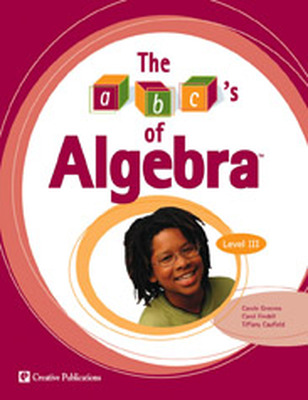# abc's of Algebra

The abc's of Algebra introduce the concept of representation as early as grade 3, and continues to develop algebraic thinking skills to make students successful with higher-level mathematics concepts.The abc's of Algebra introduces students in grades 3-7 to approachable problems that they can solve using algebraic thinking. Straightforward teaching materials clarify the "teaching moments" to move students past problem solving into symbolic representation and generalization. This early, systematic use of algebraic thinking increases students' chances of a successful transition to Algebra I!

The abc's of Algebra incorporates NCTM recommendations by addressing a wide range of algebraic thinking:

• Solving for value of unknowns
• Linear equations
• Systems of equations
• Functions
• Interpreting and applying formulas
• Linear versus nonlinear relationships

Components:

The abc's of Algebra consists of an 81-page teacher book, one book for each grade level 3-7 with 60 student pages, 10 teaching pages, 10 answer key pages. Each teacher book includes five topics. Topic organization varies by grade level to ensure exposure to algebraic thinking that is developmentally appropriate.

Sort by: